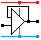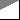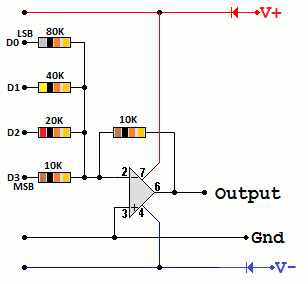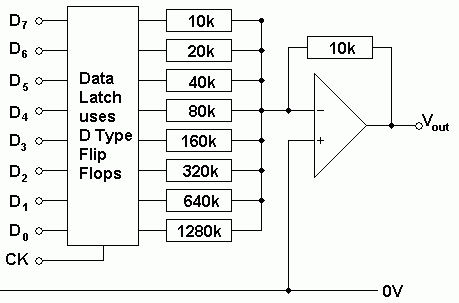Home

rOm
Quest
Glossary

Random
Page
Search
Site
Lush
Sim
Class
Subject
Images

Help
FAQ
Sign
Up
Log
In
 A Level     Signal     ADC Flash     ADC Ramp     >DAC Summing<     Distortion     Problems     Regenerator     Sample and Hold     Sampling     Serial and Parallel     signal to noise ratio

# Signal DAC Summing

Site for Eduqas/WJEC - Go to the AQA site.

A Level    SignalsQuestions 0 to 24   -->  View All

## Summing Amplifier Digital to Analogue Converter

• The input resistors have values that are in multiples of 2.
• For example 10k, 20k, 40k, 80k, 160k, 320k etc.
• This gives input gains of one, half, quarter, eighth, sixteenth, etc.
• Binary 0 or 1 values on the input are added together but the gain on each input gives the correct weighting to each bit.
• Binary 0 = 0V
• Binary 1 = 5VIn this diagram if the binary input is 0101, using the summing amplifier formula ...

Vout = -Rf ( V1 / R1 + V2 / R2 + V3 / R3 ... )

Vout = -10 ( 0 / 10 + 5 / 20 + 0 / 40 + 5 / 80 ) = 250 / 80 = -3.125 Volts

## Resolution

Resolution is the size of the voltage steps as the digital data is incremented (add one) or decremented (subtract one).

0.625 Volts is the size of the smallest step. This is the resolution of the DAC.

## Digital Ramp

If the DAC above is driven with a signal from a binary up counter increasing from 0 to 15, the output voltage will decrease in steps of 0.625 Volts.

This gives an output like this ...

## 8 Bit Latch and Summing DAC

Here is a diagram showing an 8 bit DAC with a data latch.On the rising edge of the clock pulse, the data is latched (stored in the latch).

The latch is needed if the input data is frequencly changing or not always available. When the input is valid, the rising edge of the clock causes the latch to store the data.

For example, this circuit might be connected to the data bus of a computer. Most of the time the bus is carrying data that is nothing to do with the DAC output. If the DAC is memory mapped, an address decoder would be used to trigger the clock in response to the latch's particular address.

Subject Name     Level     Topic Name     Question Heading     First Name Last Name Class ID     User ID

 CloseEscapeX Q: qNum of last_q     Q ID: Question ID         Score: num correct/num attempts         Date Done

Question Text

image url

Help Text
Debug

• You can attempt a question as many times as you like.
• If you are logged in, your first attempt, each day, is logged.
• To improve your scores, come back on future days, log in and re-do the questions that caused you problems.
• In the grade book, you can delete your answers for a topic before re-doing the questions. Avoid deleting unless you intend re-doing the questions very soon.